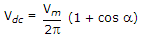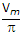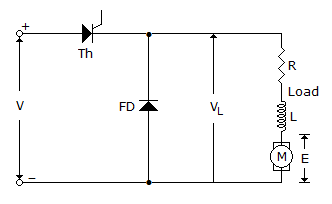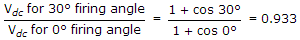# Electronics and Communication Engineering - Power Electronics

Exercise : Power Electronics - Section 1
46.
If Vdc1 is the dc output voltage in half wave controlled rectifier circuit feeding resistive load and Vdc2 is the dc output voltage in full wave controlled rectifier circuit (M - 2 connection) feeding resistive load, then
Vdc1 = 0.5 Vdc2 for all values of firing angle
Vdc1 = Vdc2 for all values of firing angle
Vdc1 ≤ 0.5 Vdc2 for all values of firing angle
Vdc1 ≥ 0.5 Vdc2 for all values of firing angle
Explanation:and Vdc =(1 + cos a).

47.
A semi converter operates in two quadrants.
True
False
Explanation:

48.
Figure shows a chopper feeding RLE load, The free wheeling diode conducts whenthyristor is on
thyristor is off
both when thyristor is on and thyristor is off
partly when thyristor is off and partly when thyristor is on
Explanation:

FD is forward biased only when thyristor is off.

49.

Assertion (A): An ideal rectifier should have unity harmonic factor

Reason (R): An ideal rectifier should have unity displacement factor.

Both A and R are correct and R is correct explanation of A
Both A and R correct but R is not correct explanation of A
A is correct but R is wrong
A is wrong but R is correct
Explanation:

Ideal rectifier should have zero harmonic factor and unity displacement factor.

50.
A single phase half wave rectifier is feeding a resistive load. When a = 0, dc output voltage is 127 V. When a = 30°, the dc output voltage will be about
127 V
120 V
110 V
100 V
Using Eq= 0.933.